Nikola Janjušević

# Supplemental material for CDLNet: Noise-Adaptive Convolutional Dictionary Learning Network for Blind Denoising and Demosaicing

## Analysis and Synthesis dictionaries ($\bm{A}^{(k)}, \, \bm{B}^{(k)}$)

How do the analysis and synthesis filters of CDLNet change over layers? Below we look at the analysis $\bm{A}^{(k)}$ and synthesis dictionaries $\bm{B}^{(k)}$ over the network layers, as well as the final synthesis dictionary $\bm{D}$. Networks with (CDLNet) and without (CDLNet-B) adaptive thresholds are shown.
CDLNet trained on noise-level range [20,30]. Analysis (A) (left) Synthesis (B) (middle) dictionaries of each layer.(right) Final synthesis dictionary (D).
CDLNet-B trained on noise-level range [20,30]. Analysis (A) (left) Synthesis (B) (middle) dictionaries of each layer.(right) Final synthesis dictionary (D).

As we progress through the layers of the network, the analysis and synthesis dictionaries look more Gabor-like and converge closer to the final dictionary. Interestingly, the very first few layers of the network also show Gabor-like structures, in contrast to the more "noisy" filters in the intermediate layers.

Further, we do not observe a significant difference between the dictionaries of CDLNet and CDLNet-B, suggesting that the generalization capability of CDLNet is solely a result of the noise-adaptive thresholds and not the learned intermediate representations.

## Learned Thresholds

How do the learned thresholds of CDLNet change over layers and subbands? For the adaptive model, we have an affine relationship with the input noise-level ($\tau^{(k)} = \tau^{(k)}_0 + \tau^{(k)}_1 \sigma$). For visualization purposes, we look at the thresholds for an input noise-level of $\sigma=25$. We also show the thresholds of an equivalent model trained without adaptive thresholds (CDLNet-B).
CDLNet trained on noise-level range [20,30]. Thresholds vary with noise-level.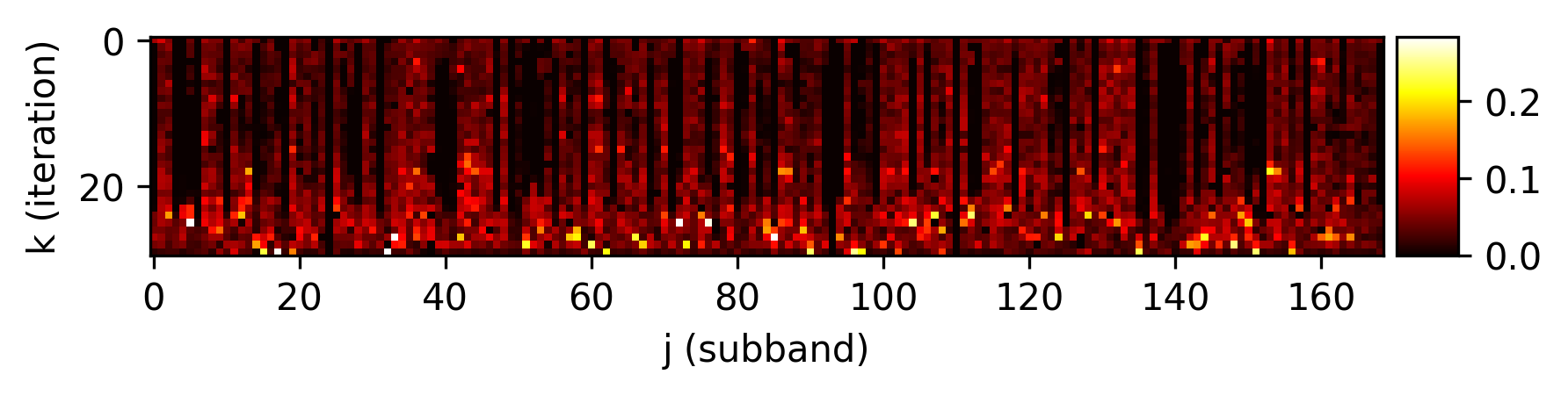CDLNet-B trained on noise-level range [20,30]. Thresholds do not vary with noise-level.

Note that the colorbars are not matched between the above two figures. For both adaptive and non-adaptive models, we see a general trend of thresholds increasing towards the final layers.

## Sparse codes ($\bm{z}^{(k)}$) over layers

How do the sparse codes of an input image vary over layers? Below we show the magnitude of the sparse codes (in layer $k$) for the cameraman test image, for an input noise-level of $\sigma=25$.
CDLNet trained on noise-level range [20,30]. Sparse codes (z) of cameraman image (noise-level 25).

We observe that the representation becomes sparse in the final layers of the network, corresponding with the higher learned thresholds. Note that sparsity is not explicitly asked for during training (there is no sparsity penalty in the loss function), but rather it is encoded through the use of the shrinkage-thresholding non-linearity (derived from the basis-pursuit denoising formulation of the network).